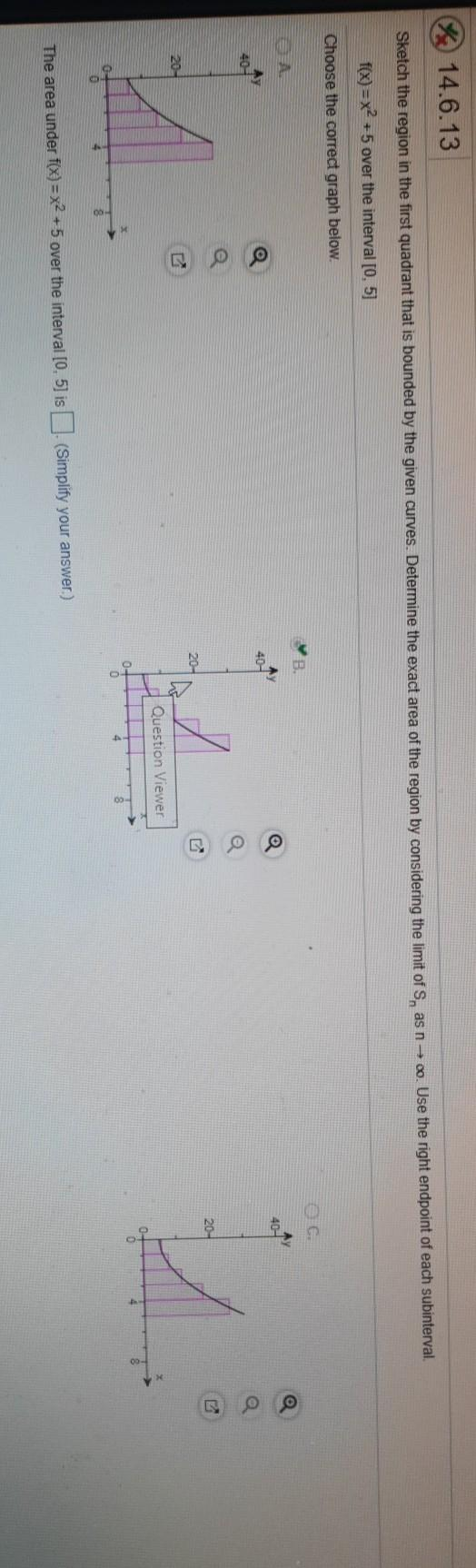### Create an Account

Home / Questions / 14.6.13 Sketch the region in the first quadrant that is bounded by the given curves. Deter...

# 14.6.13 Sketch the region in the first quadrant that is bounded by the given curves. Determine the exact area of the region by considering the limit of S, as n → 00. Use the right endpoint of each sub

14.6.13 Sketch the region in the first quadrant that is bounded by the given curves. Determine the exact area of the region by considering the limit of S, as n → 00. Use the right endpoint of each subinterval f(x) = x2 +5 over the interval [0, 5] Choose the correct graph below. AY 40- 40 20- 20- 20- d Question Viewer 0 The area under f(x)= x2 + 5 over the interval [0.5) is (Simplify your answer.)Apr 15 2021 View more View LessSubscribe To Get Solution